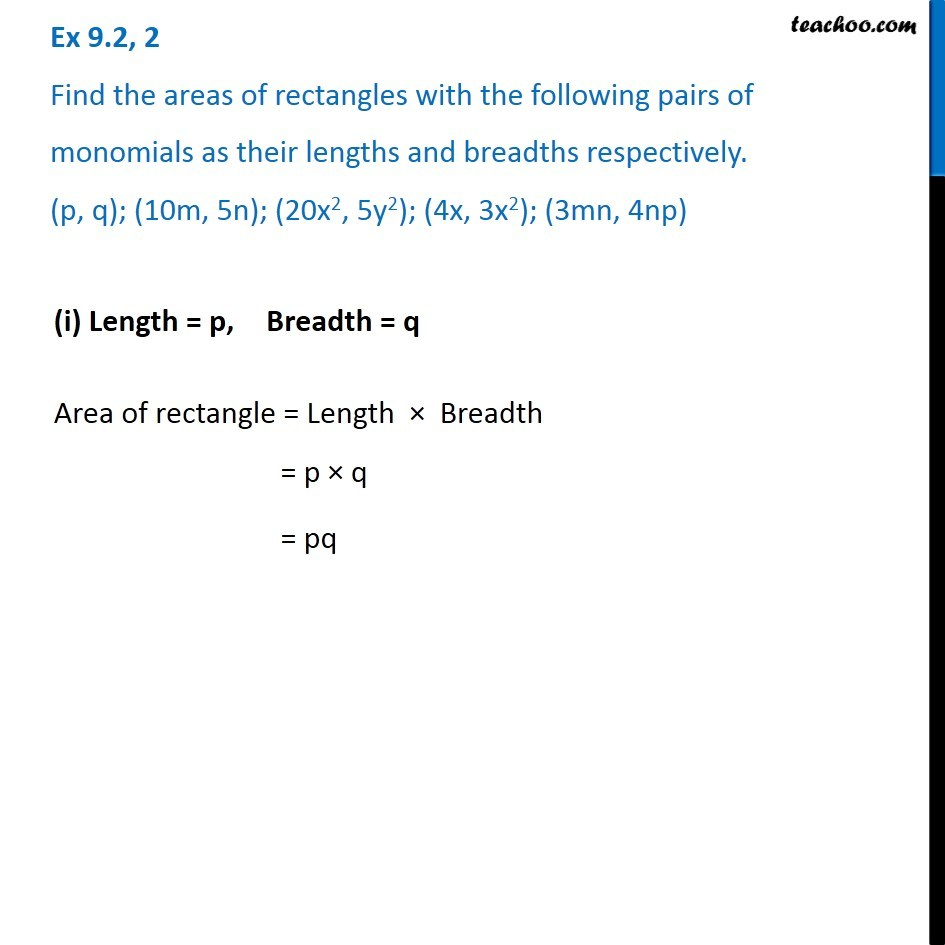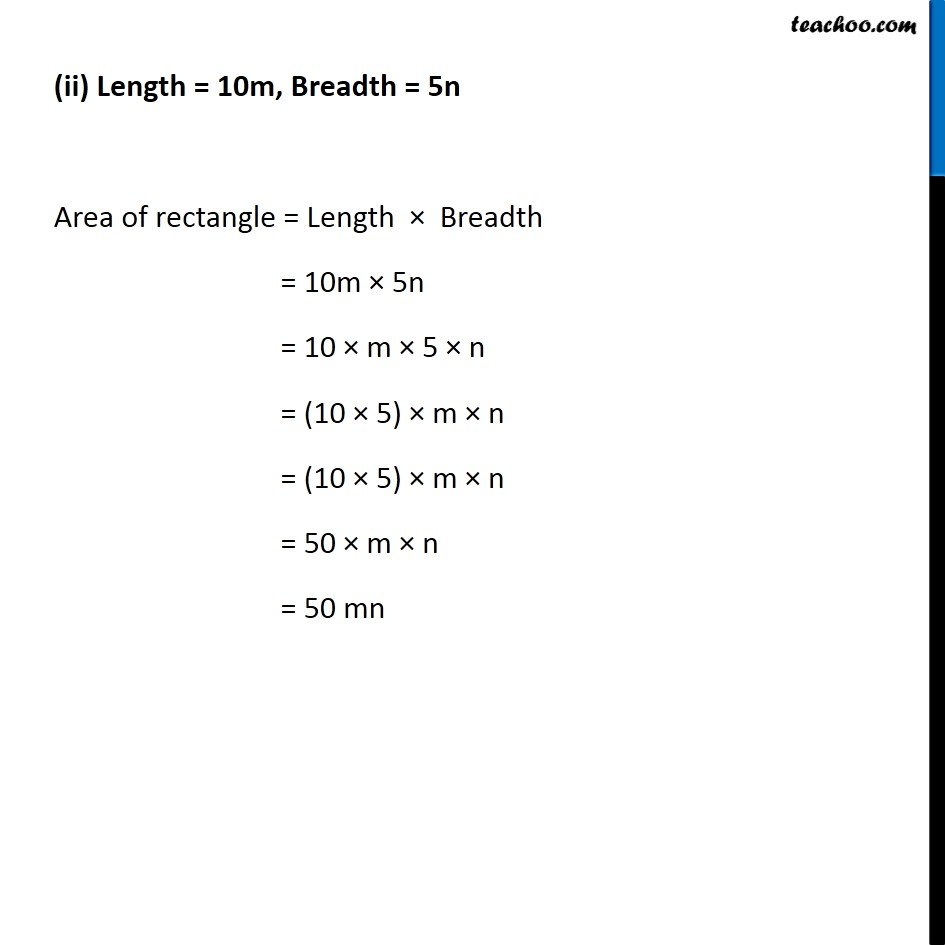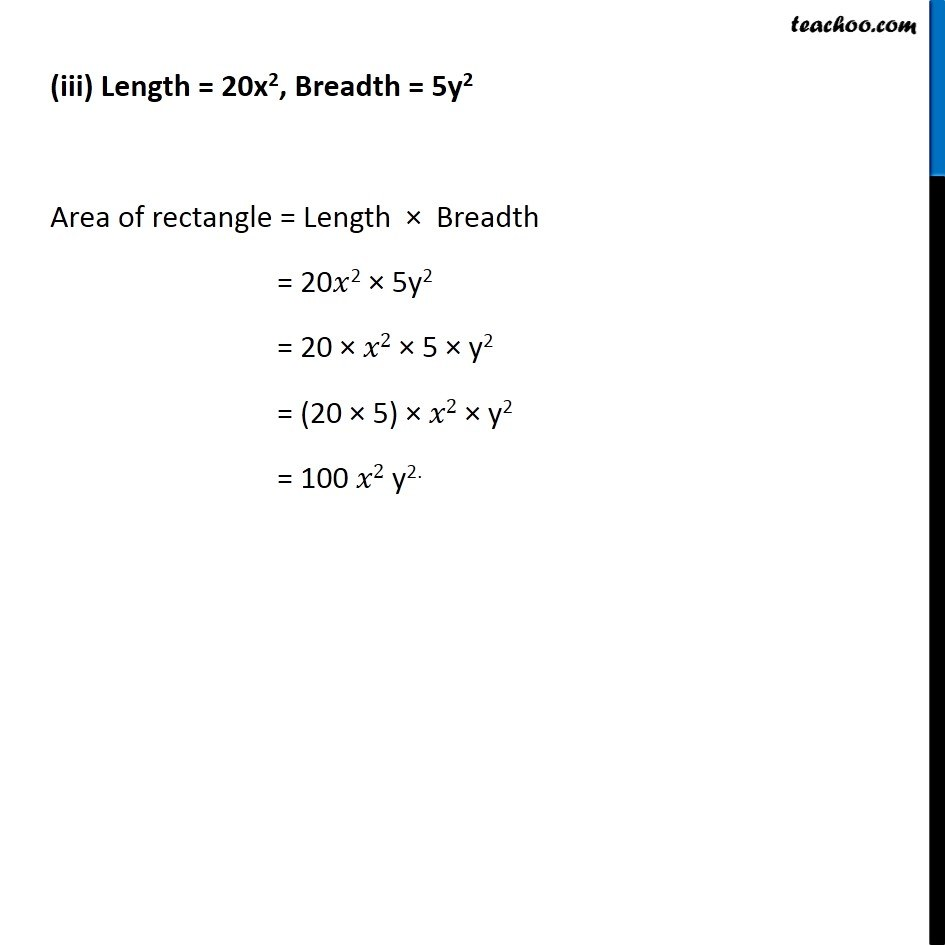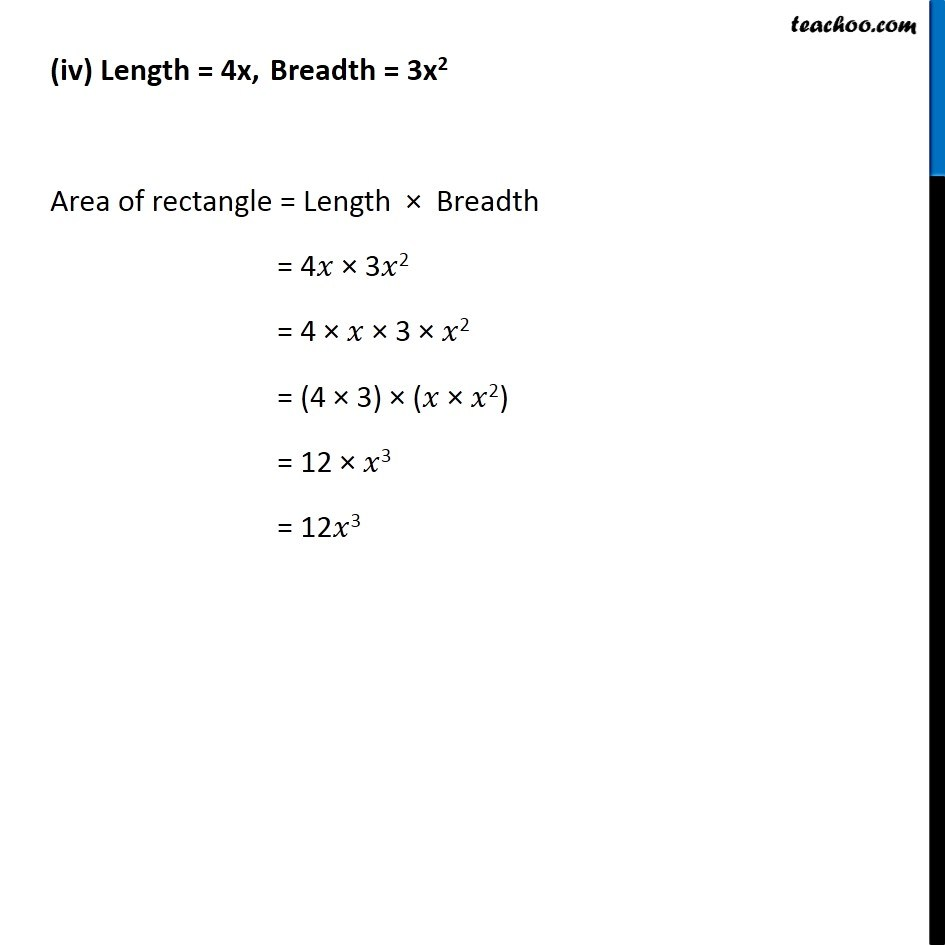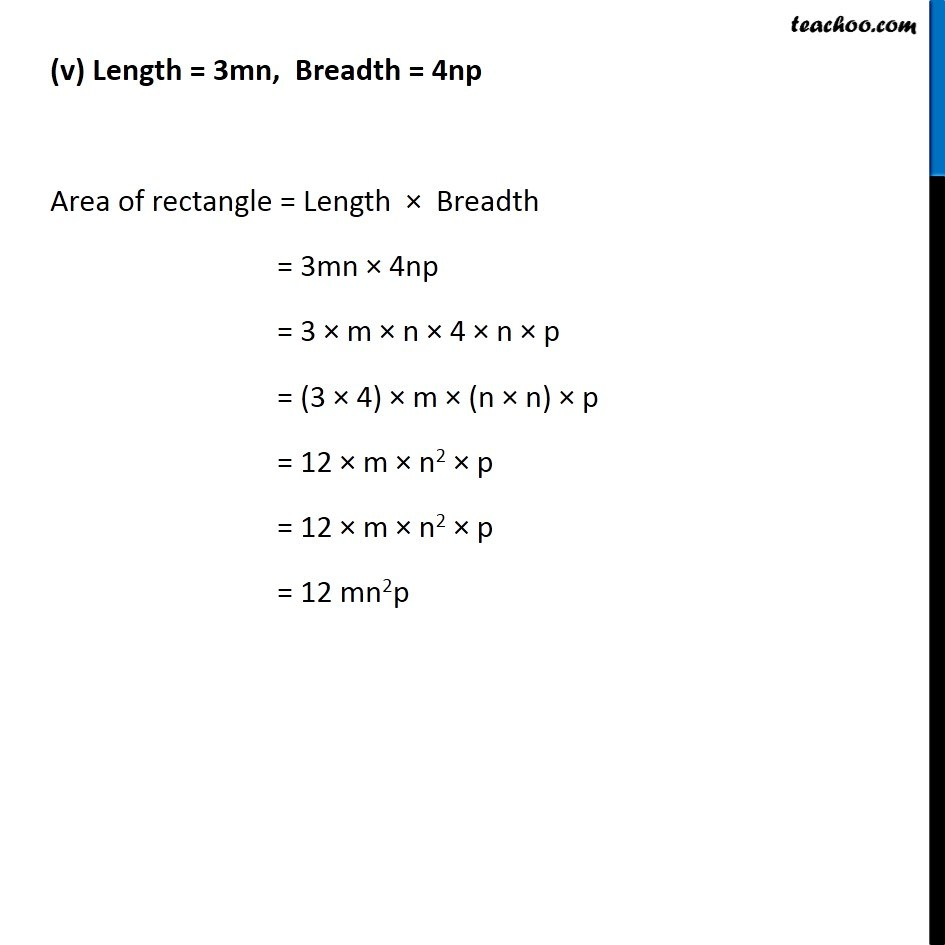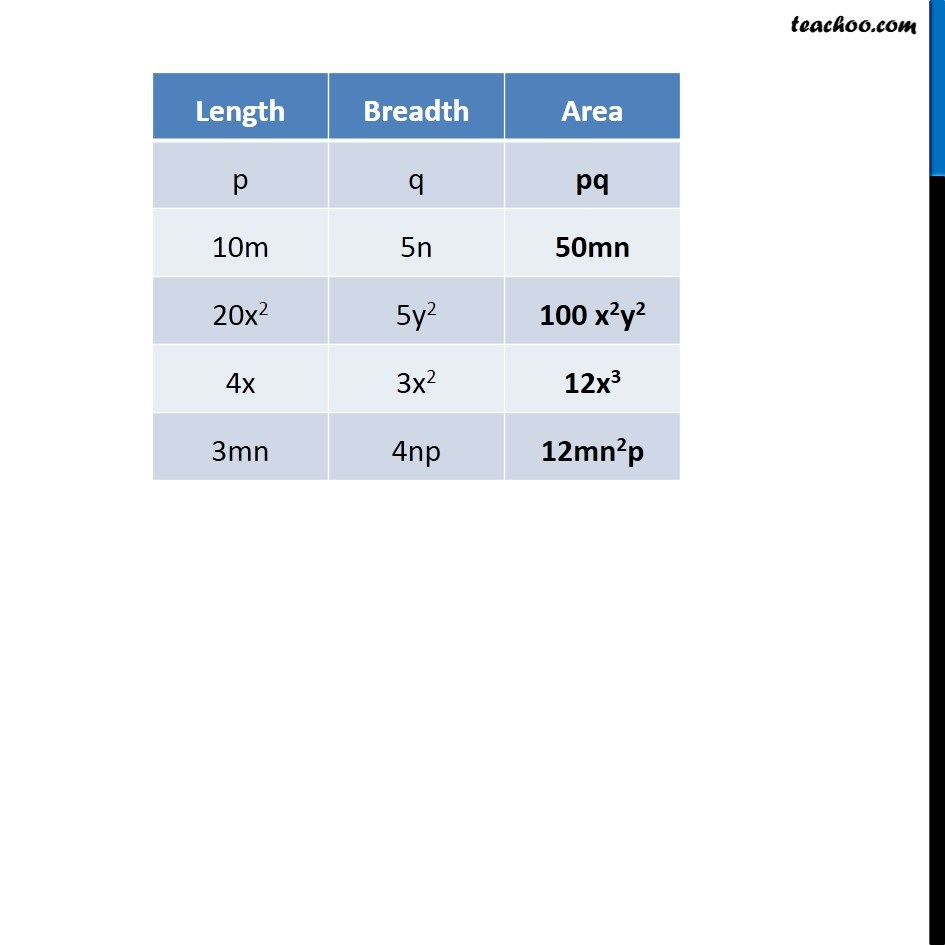1. Chapter 9 Class 8 Algebraic Expressions and Identities
2. Serial order wise
3. Ex 9.2

Transcript

Ex 9.2, 2 Find the areas of rectangles with the following pairs of monomials as their lengths and breadths respectively. (p, q); (10m, 5n); (20x2, 5y2); (4x, 3x2); (3mn, 4np) (i) Length = p, Breadth = q Area of rectangle = Length × Breadth = p × q = pq (ii) Length = 10m, Breadth = 5n Area of rectangle = Length × Breadth = 10m × 5n = 10 × m × 5 × n = (10 × 5) × m × n = (10 × 5) × m × n = 50 × m × n = 50 mn (iii) Length = 20x2, Breadth = 5y2 Area of rectangle = Length × Breadth = 20𝑥2 × 5y2 = 20 × 𝑥2 × 5 × y2 = (20 × 5) × 𝑥2 × y2 = 100 𝑥2 y2. (iv) Length = 4x, Breadth = 3x2 Area of rectangle = Length × Breadth = 4𝑥 × 3𝑥2 = 4 × 𝑥 × 3 × 𝑥2 = (4 × 3) × (𝑥 × 𝑥2) = 12 × 𝑥3 = 12𝑥3 (v) Length = 3mn, Breadth = 4np Area of rectangle = Length × Breadth = 3mn × 4np = 3 × m × n × 4 × n × p = (3 × 4) × m × (n × n) × p = 12 × m × n2 × p = 12 × m × n2 × p = 12 mn2p